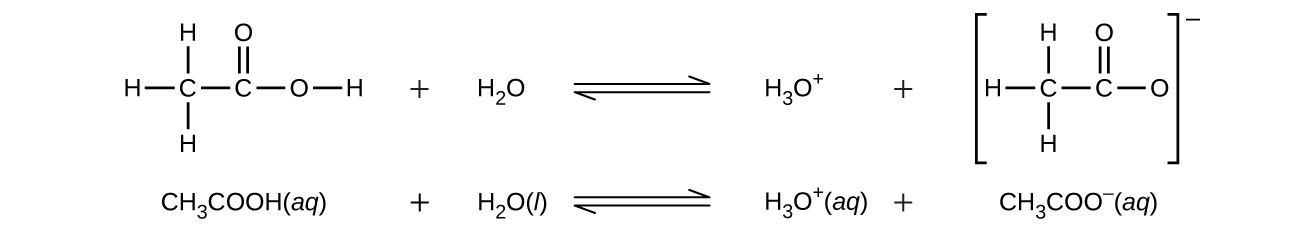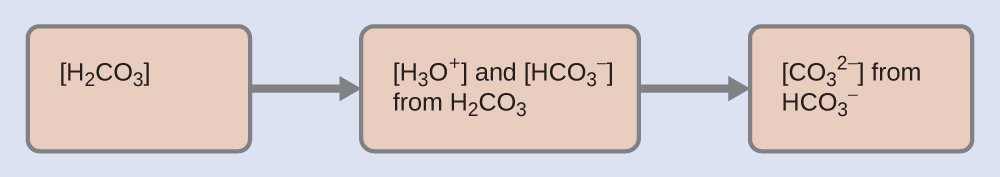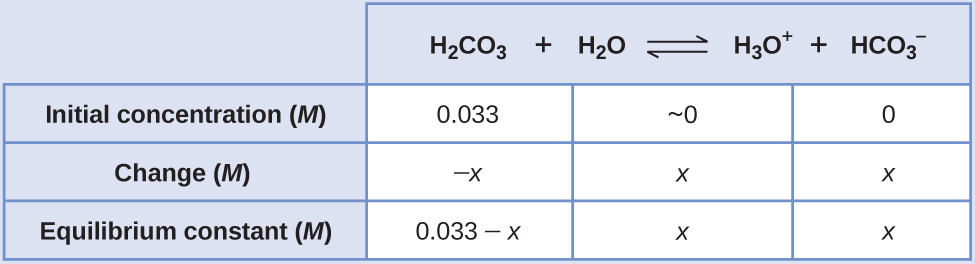Chapter 14. Acid-Base Equilibria

# 14.5 Polyprotic Acids

### Learning Objectives

By the end of this section, you will be able to:
• Extend previously introduced equilibrium concepts to acids and bases that may donate or accept more than one proton

We can classify acids by the number of protons per molecule that they can give up in a reaction. Acids such as HCl, HNO3, and HCN that contain one ionizable hydrogen atom in each molecule are called monoprotic acids. Their reactions with water are:

$\begin{array}{r @{{}\longrightarrow{}} l} \text{HCl}(aq)\;+\;\text{H}_2\text{O}(l) & \text{H}_3\text{O}^{+}(aq)\;+\;\text{Cl}^{-}(aq) \\[0.5em] \text{HNO}_3(aq)\;+\;\text{H}_2\text{O}(l) & \text{H}_3\text{O}^{+}(aq)\;+\;\text{NO}_3^{\;\;-}(aq) \\[0.5em] \text{HCN}(aq)\;+\;\text{H}_2\text{O}(l) & \text{H}_3\text{O}^{+}(aq)\;+\;\text{CN}^{-}(aq) \end{array}$

Even though it contains four hydrogen atoms, acetic acid, CH3CO2H, is also monoprotic because only the hydrogen atom from the carboxyl group (COOH) reacts with bases:Similarly, monoprotic bases are bases that will accept a single proton.

Diprotic acids contain two ionizable hydrogen atoms per molecule; ionization of such acids occurs in two steps. The first ionization always takes place to a greater extent than the second ionization. For example, sulfuric acid, a strong acid, ionizes as follows:

$\begin{array}{lr @{{}\rightleftharpoons{}} ll} \text{First\;ionization:} & \text{H}_2\text{SO}_4(aq)\;+\;\text{H}_2\text{O}(l) & \text{H}_3\text{O}^{+}(aq)\;+\;\text{HSO}_4^{\;\;-}(aq) & K_{\text{a}1} = \text{more\;than}\;10^2\text{;\;complete\;dissociation} \\[0.5em] \text{Second\;ionization:} & \text{HSO}_4^{\;\;-}(aq)\;+\;\text{H}_2\text{O}(l) & \text{H}_3\text{O}^{+}(aq)\;+\;\text{SO}_4^{\;\;2-}(aq) & K_{\text{a}2} = 1.2\;\times\;10^{-2} \end{array}$

This stepwise ionization process occurs for all polyprotic acids. When we make a solution of a weak diprotic acid, we get a solution that contains a mixture of acids. Carbonic acid, H2CO3, is an example of a weak diprotic acid. The first ionization of carbonic acid yields hydronium ions and bicarbonate ions in small amounts.

$\begin{array}{ll} \text{First\;ionization:} & \\[0.5em] \text{H}_2\text{CO}_3(aq)\;+\;\text{H}_2\text{O}(l)\;{\rightleftharpoons}\;\text{H}_3\text{O}^{+}(aq)\;+\;\text{HCO}_3^{\;\;-}(aq) & K_{\text{H}_2\text{CO}_3} = \frac{[\text{H}_3\text{O}^{+}][\text{HCO}_3^{\;\;-}]}{[\text{H}_2\text{CO}_3]} = 4.3\;\times\;10^{-7} \end{array}$

The bicarbonate ion can also act as an acid. It ionizes and forms hydronium ions and carbonate ions in even smaller quantities.

$\begin{array}{ll} \text{Second\;ionization:} & \\[0.5em] \text{HCO}_3^{\;\;-}(aq)\;+\;\text{H}_2\text{O}(l)\;{\rightleftharpoons}\;\text{H}_3\text{O}^{+}(aq)\;+\;\text{CO}_3^{\;\;2-}(aq) & K_{\text{HCO}_3^{\;\;-}} = \frac{[\text{H}_3\text{O}^{+}][\text{CO}_3^{\;\;2-}]}{[\text{HCO}_3^{\;\;-}]} = 5.6\;\times\;10^{-11} \end{array}$

$K_{\text{H}_2\text{CO}_3}$ is larger than $K_{\text{HCO}_3^{\;\;-}}$ by a factor of 104, so H2CO3 is the dominant producer of hydronium ion in the solution. This means that little of the $\text{HCO}_3^{\;\;-}$ formed by the ionization of H2CO3 ionizes to give hydronium ions (and carbonate ions), and the concentrations of H3O+ and $\text{HCO}_3^{\;\;-}$ are practically equal in a pure aqueous solution of H2CO3.

If the first ionization constant of a weak diprotic acid is larger than the second by a factor of at least 20, it is appropriate to treat the first ionization separately and calculate concentrations resulting from it before calculating concentrations of species resulting from subsequent ionization. This can simplify our work considerably because we can determine the concentration of H3O+ and the conjugate base from the first ionization, then determine the concentration of the conjugate base of the second ionization in a solution with concentrations determined by the first ionization.

### Example 1

Ionization of a Diprotic Acid
When we buy soda water (carbonated water), we are buying a solution of carbon dioxide in water. The solution is acidic because CO2 reacts with water to form carbonic acid, H2CO3. What are $[\text{H}_3\text{O}^{+}]$, $[\text{HCO}_3^{\;\;-}]$, and $[\text{CO}_3^{\;\;2-}]$ in a saturated solution of CO2 with an initial [H2CO3] = 0.033 M?

$\begin{array}{r @{{}\rightleftharpoons{}} ll} \text{H}_2\text{CO}_3(aq)\;+\;\text{H}_2\text{O}(l) & \text{H}_3\text{O}^{+}(aq)\;+\;\text{HCO}_3^{\;\;-}(aq) & K_{\text{a}1} = 4.3\;\times\;10^{-7} \\[0.5em] \text{HCO}_3^{\;\;-}(aq)\;+\;\text{H}_2\text{O}(l) & \text{H}_3\text{O}^{+}(aq)\;+\;\text{CO}_3^{\;\;2-}(aq) & K_{\text{a}2} = 5.6\;\times\;10^{-11} \end{array}$

Solution
As indicated by the ionization constants, H2CO3 is a much stronger acid than $\text{HCO}_3^{\;\;-}$, so H2CO3 is the dominant producer of hydronium ion in solution. Thus there are two parts in the solution of this problem: (1) Using the customary four steps, we determine the concentration of H3O+ and $\text{HCO}_3^{\;\;-}$ produced by ionization of H2CO3. (2) Then we determine the concentration of $\text{CO}_3^{\;\;2-}$ in a solution with the concentration of H3O+ and $\text{HCO}_3^{\;\;-}$ determined in (1). To summarize:1. Determine the concentrations of $\text{H}_3\text{O}^{+}$ and $\text{HCO}_3^{\;\;-}$.
$\text{H}_2\text{CO}_3(aq)\;+\;\text{H}_2\text{O}(l)\;{\rightleftharpoons}\;\text{H}_3\text{O}^{+}(aq)\;+\;\text{HCO}_3^{\;\;-}(aq)\;\;\;\;\;\;\;K_{\text{a}1} = 4.3\;\times\;10^{-7}$

As for the ionization of any other weak acid:An abbreviated table of changes and concentrations shows:Substituting the equilibrium concentrations into the equilibrium gives us:

$K_{\text{H}_2\text{CO}_3} = \frac{[\text{H}_3\text{O}^{+}][\text{HCO}_3^{\;\;-}]}{[\text{H}_2\text{CO}_3]} = \frac{(x)(x)}{0.033\;-\;x} = 4.3\;\times\;10^{-7}$

Solving the preceding equation making our standard assumptions gives:

$x = 1.2\;\times\;10^{-4}$

Thus:

$[\text{H}_2\text{CO}_3] = 0.033\;M$
$[\text{H}_3\text{O}^{+}] = [\text{HCO}_3^{\;\;-}] = 1.2\;\times\;10^{-4}\;M$
2. Determine the concentration of $\text{CO}_3^{\;\;2-}$ in a solution at equilibrium with $[\text{H}_3\text{O}^{+}]$and $[\text{HCO}_3^{\;\;-}]$both equal to 1.2 ×10−4 M.
$\text{HCO}_3^{\;\;-}(aq)\;+\;\text{H}_2\text{O}(l)\;{\rightleftharpoons}\;\text{H}_3\text{O}^{+}(aq)\;+\;\text{CO}_3^{\;\;2-}(aq)$
$K_{\text{HCO}_3^{\;\;-}} = \frac{[\text{H}_3\text{O}^{+}][\text{CO}_3^{\;\;2-}]}{[\text{HCO}_3^{\;\;-}]} = \frac{(1.2\;\times\;10^{-4})[\text{CO}_3^{\;\;2-}]}{1.2\;\times\;10^{-4}}$
$[\text{CO}_3^{\;\;2-}] = \frac{(5.6\;\times\;10^{-11})(1.2\;\times\;10^{-4})}{1.2\;\times\;10^{-4}} = 5.6\;\times\;10^{-11}\;M$

To summarize: In part 1 of this example, we found that the H2CO3 in a 0.033-M solution ionizes slightly and at equilibrium [H2CO3] = 0.033 M; $[\text{H}_3\text{O}^{+}] = 1.2\;\times\;10^{-4}$; and $[\text{HCO}_3^{\;\;-}] = 1.2\;\times\;10^{-4}\;M$. In part 2, we determined that $[\text{CO}_3^{\;\;2-}] = 5.6\;\times\;10^{-11}\;M$.

The concentration of H2S in a saturated aqueous solution at room temperature is approximately 0.1 M. Calculate [H3O+], [HS], and [S2−] in the solution:

$\begin{array}{r @{{}\rightleftharpoons{}} ll} \text{H}_2\text{S}(aq)\;+\;\text{H}_2\text{O}(l) & \text{H}_3\text{O}^{+}(aq)\;+\;\text{HS}^{-}(aq) & K_{\text{a}1} = 8.9\;\times\;10^{-8} \\[0.5em] \text{HS}^{-}(aq)\;+\;\text{H}_2\text{O}(l) & \text{H}_3\text{O}^{+}(aq)\;+\;\text{S}_2^{\;\;-}(aq) & K_{\text{a}2} = 1.0\;\times\;10^{-19} \end{array}$

[H2S] = 0.1 M; $[\text{H}_3\text{O}^{+}]$ = [HS] = 0.000094 M; [S2−] = 1 × 10−19M

We note that the concentration of the sulfide ion is the same as Ka2. This is due to the fact that each subsequent dissociation occurs to a lesser degree (as acid gets weaker).

A triprotic acid is an acid that has three dissociable protons that undergo stepwise ionization: Phosphoric acid is a typical example:

$\begin{array}{lr @{{}\rightleftharpoons{}} ll} \text{First\;ionization:} & \text{H}_3\text{PO}_4(aq)\;+\;\text{H}_2\text{O}(l) & \text{H}_3\text{O}^{+}(aq)\;+\;\text{H}_2\text{PO}_4^{\;\;-}(aq) & K_{\text{a}1} = 7.5\;\times\;10^{-3} \\[0.5em] \text{Second\;ionization:} & \text{H}_2\text{PO}_4^{\;\;-}(aq)\;+\;\text{H}_2\text{O}(l) & \text{H}_3\text{O}^{+}(aq)\;+\;\text{HPO}_4^{\;\;2-}(aq) & K_{\text{a}2} = 6.2\;\times\;10^{-8} \\[0.5em] \text{Third\;ionization:} & \text{HPO}_4^{\;\;2-}(aq)\;+\;\text{H}_2\text{O}(l) & \text{H}_3\text{O}^{+}(aq)\;+\;\text{PO}_4^{\;\;3-}(aq) & K_{\text{a}3} = 4.2\;\times\;10^{-13} \end{array}$

As with the diprotic acids, the differences in the ionization constants of these reactions tell us that in each successive step the degree of ionization is significantly weaker. This is a general characteristic of polyprotic acids and successive ionization constants often differ by a factor of about 105 to 106.

This set of three dissociation reactions may appear to make calculations of equilibrium concentrations in a solution of H3PO4 complicated. However, because the successive ionization constants differ by a factor of 105 to 106, the calculations can be broken down into a series of parts similar to those for diprotic acids.

Polyprotic bases can accept more than one hydrogen ion in solution. The carbonate ion is an example of a diprotic base, since it can accept up to two protons. Solutions of alkali metal carbonates are quite alkaline, due to the reactions:

$\text{H}_2\text{O}(l)\;+\;\text{CO}_3^{\;\;2-}(aq)\;{\rightleftharpoons}\;\text{HCO}_3^{\;\;-}(aq)\;+\;\text{OH}^{-}(aq)\;\;\;\;\;\;\text{and}\;\;\;\;\;\;\text{H}_2\text{O}(l)\;+\;\text{HCO}_3^{\;\;-}(aq)\;{\rightleftharpoons}\;\text{H}_2\text{CO}_3(aq)\;+\;\text{OH}^{-}(aq)$

# Key Concepts and Summary

An acid that contains more than one ionizable proton is a polyprotic acid. The protons of these acids ionize in steps. The differences in the acid ionization constants for the successive ionizations of the protons in a polyprotic acid usually vary by roughly five orders of magnitude. As long as the difference between the successive values of Ka of the acid is greater than about a factor of 20, it is appropriate to break down the calculations of the concentrations of the ions in solution into a series of steps.

### Chemistry End of Chapter Exercises

1. Which of the following concentrations would be practically equal in a calculation of the equilibrium concentrations in a 0.134-M solution of H2CO3, a diprotic acid: $[\text{H}_3\text{O}^{+}]$, [OH], [H2CO3], $[\text{HCO}_3^{\;\;-}]$, $[\text{CO}_3^{\;\;2-}]$? No calculations are needed to answer this question.
2. Calculate the concentration of each species present in a 0.050-M solution of H2S.
3. Calculate the concentration of each species present in a 0.010-M solution of phthalic acid, C6H4(CO2H)2.
$\begin{array}{r @{{}\rightleftharpoons{}} ll} \text{C}_6\text{H}_4(\text{CO}_2\text{H})_2(aq)\;+\;\text{H}_2\text{O}(l) & \text{H}_3\text{O}^{+}(aq)\;+\;\text{C}_6\text{H}_4(\text{CO}_2\text{H})(\text{CO}_2)^{-}(aq) & K_{\text{a}} = 1.1\;\times\;10^{-3} \\[0.5em] \text{C}_6\text{H}_4(\text{CO}_2\text{H})(\text{CO}_2)(aq)\;+\;\text{H}_2\text{O}(l) & \text{H}_3\text{O}^{+}(aq)\;+\;\text{C}_6\text{H}_4(\text{CO}_2)_2^{\;\;2-}(aq) & K_{\text{a}} = 3.9\;\times\;10^{-6} \end{array}$
4. Salicylic acid, HOC6H4CO2H, and its derivatives have been used as pain relievers for a long time. Salicylic acid occurs in small amounts in the leaves, bark, and roots of some vegetation (most notably historically in the bark of the willow tree). Extracts of these plants have been used as medications for centuries. The acid was first isolated in the laboratory in 1838.

(a) Both functional groups of salicylic acid ionize in water, with Ka = 1.0 × 10−3 for the—CO2H group and 4.2 × 10−13 for the −OH group. What is the pH of a saturated solution of the acid (solubility = 1.8 g/L).

(b) Aspirin was discovered as a result of efforts to produce a derivative of salicylic acid that would not be irritating to the stomach lining. Aspirin is acetylsalicylic acid, CH3CO2C6H4CO2H. The −CO2H functional group is still present, but its acidity is reduced, Ka = 3.0 × 10−4. What is the pH of a solution of aspirin with the same concentration as a saturated solution of salicylic acid (See Part a).

(c) Under some conditions, aspirin reacts with water and forms a solution of salicylic acid and acetic acid:

$\text{CH}_3\text{CO}_2\text{C}_6\text{H}_4\text{CO}_2\text{H}(aq)\;+\;\text{H}_2\text{O}(l)\;{\longrightarrow}\;\text{HOC}_6\text{H}_4\text{CO}_2\text{H}(aq)\;+\;\text{CH}_3\text{CO}_2\text{H}(aq)$

i. Which of the acids, salicylic acid or acetic acid, produces more hydronium ions in such a solution?

ii. What are the concentrations of molecules and ions in a solution produced by the hydrolysis of 0.50 g of aspirin dissolved in enough water to give 75 mL of solution?

5. The ion HTe is an amphiprotic species; it can act as either an acid or a base.

(a) What is Ka for the acid reaction of HTe with H2O?

(b) What is Kb for the reaction in which HTe functions as a base in water?

(c) Demonstrate whether or not the second ionization of H2Te can be neglected in the calculation of [HTe] in a 0.10 M solution of H2Te.

## Glossary

diprotic acid
acid containing two ionizable hydrogen atoms per molecule. A diprotic acid ionizes in two steps
diprotic base
base capable of accepting two protons. The protons are accepted in two steps
monoprotic acid
acid containing one ionizable hydrogen atom per molecule
stepwise ionization
process in which an acid is ionized by losing protons sequentially
triprotic acid
acid that contains three ionizable hydrogen atoms per molecule; ionization of triprotic acids occurs in three steps

### Solutions

Answers to Chemistry End of Chapter Exercises

1. [H3O+] and $[\text{HCO}_3^{\;\;-}]$ are practically equal

3. [C6H4(CO2H)2] 7.2 × 10−3M, [C6H4(CO2H)(CO2)] = [H3O+] 2.8 × 10−3M, $[\text{C}_6\text{H}_4(\text{CO}_2)_2^{\;\;2-}]$ 3.9 × 10−6M, [OH] 3.6 × 10−12M

5. (a) $K_{\text{a}2} = 1.5\;\times\;10^{-11}$;

(b) $K_{\text{b}} = 4.3\;\times\;10^{-12}$;

(c) $\frac{[\text{Te}^{2-}][\text{H}_3\text{O}^{+}]}{[\text{HTe}^{-}]} = \frac{(x)(0.0141\;+\;x)}{(0.0141\;-\;x)}\;{\approx}\;\frac{(x)(0.0141)}{0.0141} = 1.5\;\times\;10^{-11}$

Solving for x gives 1.5 × 10−11M. Therefore, compared with 0.014 M, this value is negligible (1.1 × 10−7%).